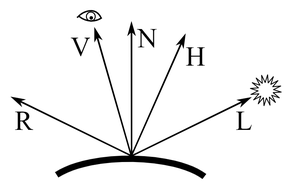## 冯氏光照模型（Phong reflection model）

• 环境光（Ambient Light）：用于模拟物体周围环境固有存在的光线；通常是阳光，月光这类光线对物体造成漫反射光，在物体间不停地反射，达到某种程度恒定的光线；
• 漫反射光（Diffuse Light）：用于模拟光线照射到粗糙表面形成的反射光；
• 镜面反射光（Specular Light）：用于模拟光线照射到光滑表面形成的亮点（即高光）；### 环境光的计算

$L_{环境} = L_{光源} * K_{环境}$

### 漫反射的计算

$K_{漫反射} = max(D_{光源} \cdot N,\ 0.0)\\ L_{漫反射} = L_{光源} * K_{漫反射}$

### 镜面反射光的计算

$K_{镜面反射} = max(V \cdot R,\ 0.0)\\ L_{镜面反射} = L_{光源} * K_{镜面反射}$

$K_{镜面反射} = max((V \cdot R)^{shininess},\ 0.0)$

$L_{反射} = L_{环境} + L_{漫反射} + L_{镜面反射}\\ Color_{反射} = Color_{物体} * L_{反射}$

## Blinn–Phong 光照模型

Blinn–Phong光照模型实际上是对于冯氏光照模型的一种改进，主要区别就在于镜面反射因子的计算；

### 改进后的镜面反射因子

Blinn–Phong光照模型中，镜面反射因子是由光源方向（L）和观察方向（V）的半程向量H）与法向量（N）之间的点积构成的；

$K_{镜面反射} = max((H \cdot N)^{shininess},\ 0.0)$